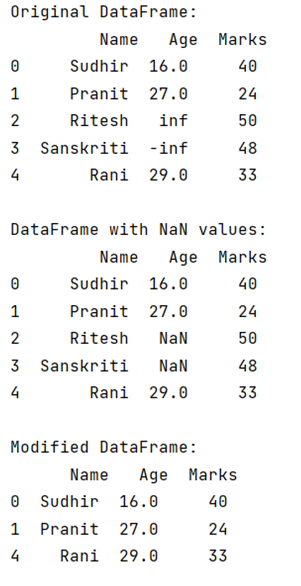# How to drop infinite values from DataFrames in Pandas?

Given a DataFrame, we have to drop infinite values from it.
Submitted by Pranit Sharma, on May 11, 2022

Pandas is a special tool that allows us to perform complex manipulations of data effectively and efficiently. Inside pandas, we mostly deal with a dataset in the form of DataFrame. DataFrames are 2-dimensional data structures in pandas. DataFrames consist of rows, columns, and the data. DataFrame can be created with the help of python dictionaries or lists but in the real world, CSV files are imported and then converted into DataFrames. Inside DataFrame, certain values can not be analyzed or operated to analyze the data efficiently.

Here, we are going to learn how to drop infinite values in DataFrame? For this purpose, first, we will replace all the infinite values with NaN, and then we will drop all the NaN values.

## pandas.DataFrame.replace() Method

The pandas.DataFrame.replace() method in Pandas is a simple method that takes two parameters, first is the value of the string, list, dictionary, etc which has to be replaced. Secondly, it takes the value with which our data has to be replaced.

## pandas.DataFrame.drop() Method

This method is used to remove a specified row or column from the pandas DataFrame. Since rows and columns are based on index and axis values respectively, by passing index or axis value inside pandas.DataFrame.drop() method we can delete that particular row or column.

To work with pandas, we need to import pandas package first, below is the syntax:

```import pandas as pd
```

Let us understand with the help of an example:

```# Importing pandas package
import pandas as pd

# Importing numpy package
import numpy as np

# Create a dictionary for the dataframe
dict = {
'Name': ['Sudhir', 'Pranit', 'Ritesh','Sanskriti', 'Rani'],
'Age': [16, 27, np.inf, -np.inf, 29],
'Marks': [40, 24, 50, 48, 33]
}

# Converting Dictionary to Pandas Dataframe
df = pd.DataFrame(dict)

# Display original DataFrame
print("Original DataFrame:\n",df,"\n")

# Replacing infinite with nan
df.replace([np.inf, -np.inf], np.nan, inplace=True)

# Display df with NaN values
print("DataFrame with NaN values:\n",df,"\n")

# Dropping the rows with nan values
df.dropna(inplace=True)

# Display Df
print("Modified DataFrame:\n",df)
```

Output:Preparation